# Yieldbot Launches Vizard for Data Visualization

Happy New Year! To usher in 2016, Yieldbot is happy to announce the open source release of Vizard, a small Clojure library for data visualization. The motivation for Vizard was to have a simple way to produce plots using Vega from a Clojure REPL. In particular, we wanted to easily do ad hoc plotting in our day-to-day work without resorting to things like R, matplotlib, or Google spreadsheets.

The data team at Yieldbot has been using the visualization grammar Vega in various internal Clojure(script) applications for a couple of years. We tend to prefer simple graphs like scatter plots and bar charts, and Vega specs for these are fairly easy to generate. It is also easy to share them with our front-end team that works in javascript.

Getting Started

`[yieldbot/vizard "0.1.0"]`

`(require '[vizard [core :refer :all] [plot :as plot]])`
`(start-plot-server!)`

This will start the vizard server and open your browser to the correct port.

Examples

Let’s go through a few examples. First we’ll generate some data to plot:

```(defn sine-data [num-points freq]
(for [i (range num-points)
:let [x (/ (* i 2 Math/PI) num-points)]]
{:x x :y (Math/sin (* freq x)) :col "sine"}))
(plot! (plot/vizard {:mark-type :scatter} (sine-data 100 1)))
```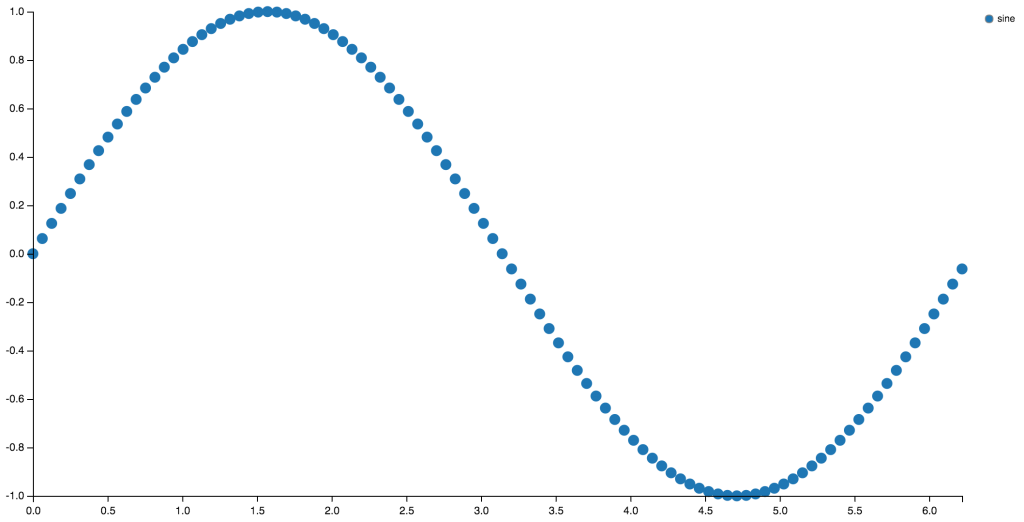We use the `vizard` multimethod to generate the spec. It takes two arguments: a config map and a sequence of data points. It dispatches on the value of the `:mark-type` key in the config map. The `plot!` function POSTs the spec to the server, which causes it to be rendered and displayed in the browser.

We can plot the same data as a line with:

`(plot! (plot/vizard {:mark-type :line} (sine-data 100 1)))`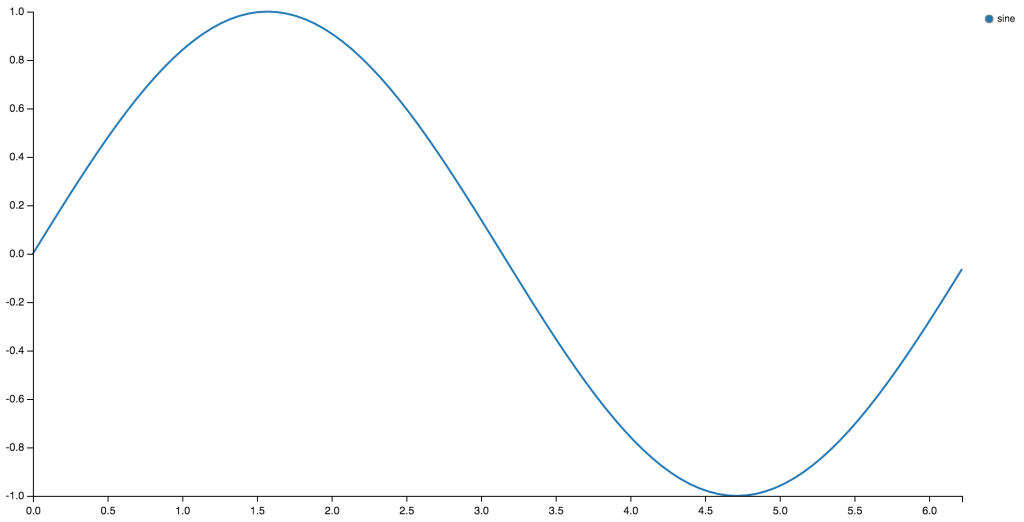Now we’ll add some noise to this sine function and plot a few functions on the same graph.

```(defn group-sine-data [num-points freq & names]
(let [points (sine-data num-points freq)
rename-w-noise (fn [n]
(for [p points]
(assoc p
:y (+ (:y p) (rand) -0.5)
:col n)))]
(mapcat rename-w-noise names)))
(plot! (plot/vizard {:mark-type :line
:encoding
{:x {:field :x :scale :linear :label "x axis"}
:y {:field :y :scale :linear :label "y axis"}
:g {:field :col}}
:color "category20b"
:legend? true}
(group-sine-data 100 1 "foo" "bar" "baz" "poot")))
```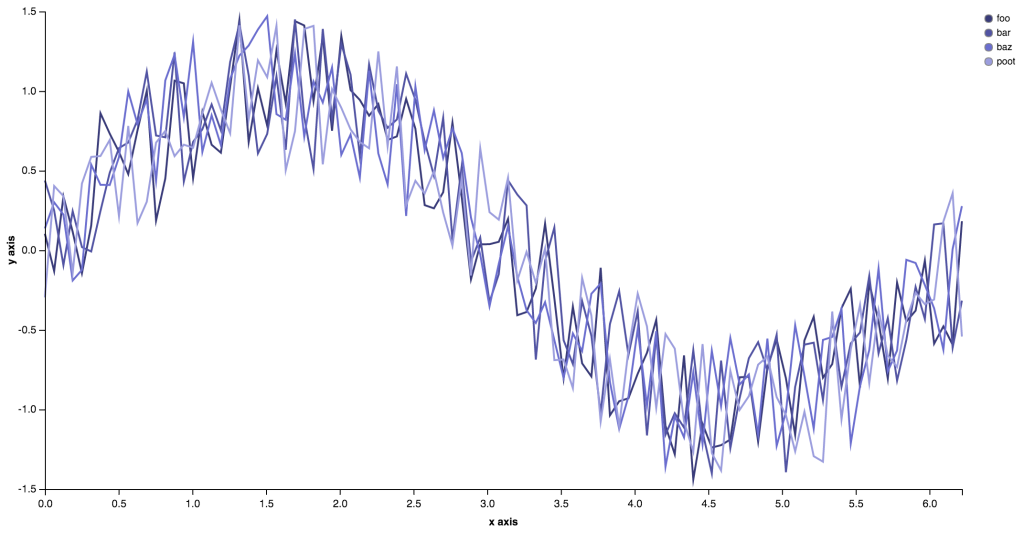Here I’ve made the options you can set in the config map explicit. The `:encoding` map allows you to specify how the data points are named and if the axes represent numerical, ordinal, or temporal scales with the keywords `:linear`, `:ordinal`, or `:time`. You can also set the color scheme (see here for categorical color options), axis labels, or whether you want a legend or not.

Currently, there are two other mark types available that generate bar and area plots:

```(defn group-data [num-points val-max & names]
(letfn [(rand-ints [name]
(for [x (range num-points)]
{:x x :y (rand-int val-max) :col name}))]
(mapcat rand-ints names)))
(plot! (plot/vizard {:mark-type :bar
:encoding {:x {:field :x :scale :ordinal}
:y {:field :y :scale :linear}
:g {:field :col}}
:color "category20b"
:legend? true}
(group-data 20 100 "foo" "bar" "baz" "poot")))
```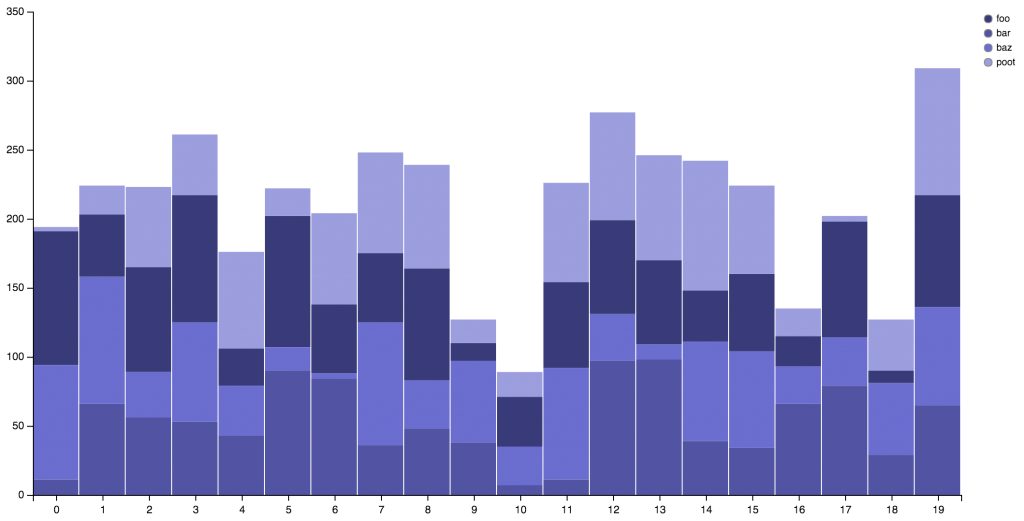```(plot! (plot/vizard {:mark-type :area
:encoding {:x {:field :x :scale :linear}
:y {:field :y :scale :linear}
:g {:field :col}}
:color "category20b"
:legend? true}
(group-data 20 100 "foo" "bar" "baz" "poot")))
```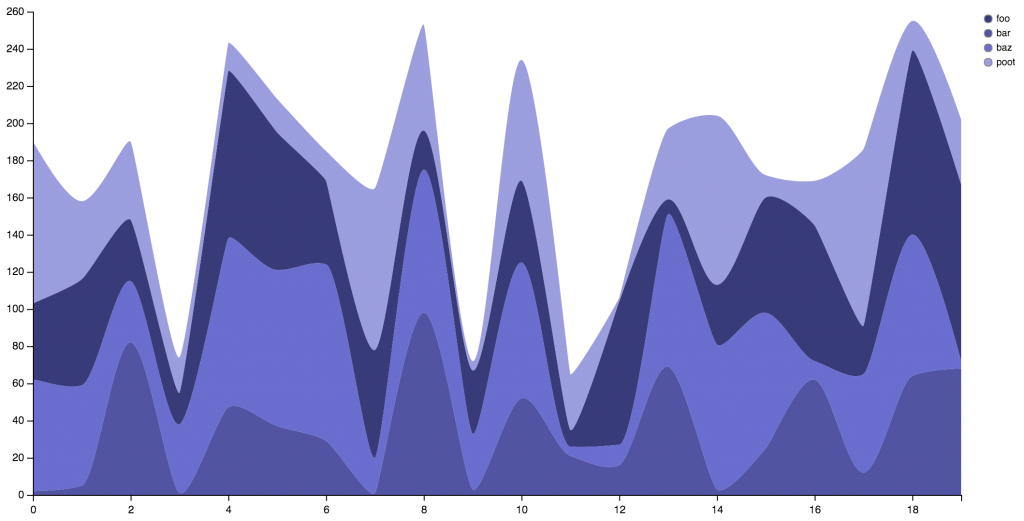Note that all of the mark types support single and multiple data series.

Going Beyond the Basic Specs

New types of plots can be generated by writing additional `vizard` methods. Alternatively, since `vizard` returns a Clojure object representing the spec, a little knowledge about how Vega specs work (see here) allows you to manipulate that spec directly to add new features or data.

For example, I recently wanted to add some confidence intervals around a line, but this isn’t something we can do with the existing methods. So let’s take the sine from the first example and add a band around it.

```(defn area-data [num-points freq delta]
(for [{:keys [x y]} (sine-data num-points freq)]
{:x x :y (+ y delta) :y2 (- y delta)}))
(-> (plot/vizard {:mark-type :line :color "category20b"}
(sine-data 100 1))
(assoc-in [:data 1] {:name :confidence
:values (area-data 100 1 0.2)})
(assoc-in [:marks 1] {:type :area
:from {:data :confidence}
:properties {:enter
{:x {:scale "x" :field :x}
:y {:scale "y" :field :y}
:y2 {:scale "y" :field :y2}
:interpolate {:value
:monotone}
:fill {:value "#666"}}
:update {:fillOpacity {:value 0.25}}}})
plot!)
```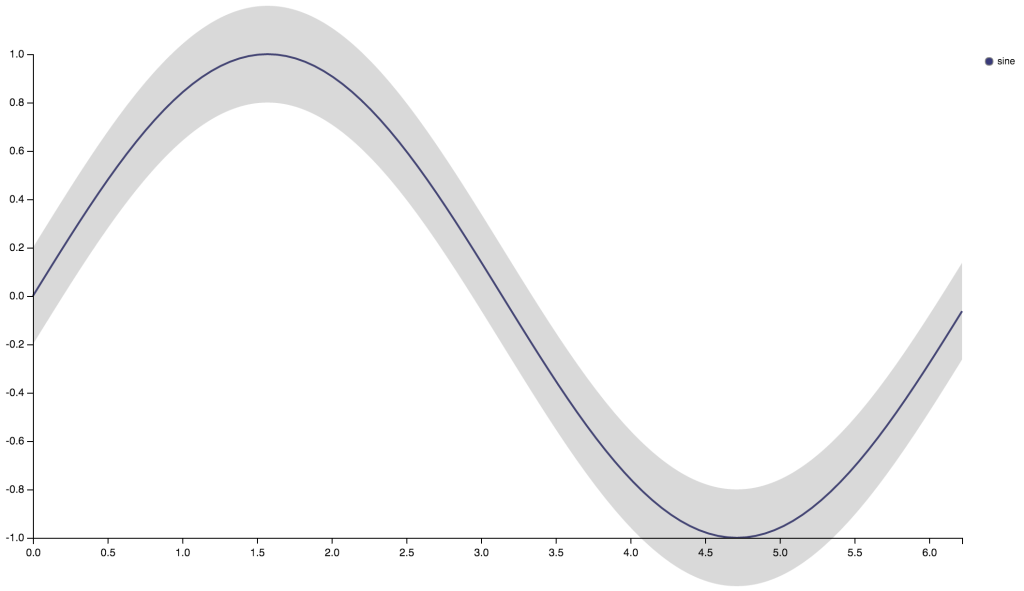We generated the line plot in the usual way, but poked some extra info into the `:data` and `:marks` sections of the spec. If you are already familiar with Vega, this gives a powerful way to manipulate Vega specs as pure Clojure datastructures.

Helper functions in the plot namespace can be used to make this a little more succinct.

```(-> (plot/vizard {:mark-type :line :color "category20b"}
(sine-data 100 1))
(assoc-in [:data 1]
(plot/d :confidence :values (area-data 100 1 0.2)))
(assoc-in [:marks 1]
(plot/mark
:area
:from (plot/from :confidence)
:properties (plot/properties
:enter [[:x :scale "x" :field :x]
[:y :scale "y" :field :y]
[:y2 :scale "y" :field :y2]
[:interpolate :value :monotone]
[:fill :value "#666"]]
:update [[:fillOpacity :value 0.25]])))
plot!)
```

We have open sourced Vizard and made it available on GitHub. We welcome pull requests for new specs and features and appreciate any comments or suggestions you may have.

__

James Dunn is a Data Scientist on the Yieldbot Engineering team and works on the machine learning behind Yieldbot’s ad serving technology